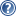###LON-CAPA Help

A tolerance parameter determines how closely the system will require the student's answer to be in order to count it correct. The tolerance will default to zero if it is not defined. The tolerance parameter should always be defined for a numerical problem unless you are certain only integer answers are generated from your script and you want students to reply with exactly that integer.

If the computer answer is a floating point number, the tolerance should not be zero. Computers can only approximate computations involving real numbers. For instance, a computer's [decimal] answer to the simple problem [ 1/3] is "0.33333333333333331". It should be an infinite series of 3's, and there certainly shouldn't be a "1" in the answer, but no computer can represent an infinitely long, infinitely detailed real number. Therefore, for any problem where the answer is not an integer, you need to allow a tolerance factor, or the students will find it nearly impossible to exactly match the computer's idea of the answer. You may find the default tolerance too large for some problems, so adjust as appropriate.

There are three kinds of tolerance. For some answer A and a tolerance T,

1. an Absolute tolerance will take anything in the range A±T. So if A=10 and T=2, then anything between 8 and 12 is acceptable. Any number in the tolerance field without a % symbol is an absolute tolerance.

2. a Relative tolerance will take anything in the range A±aT, where T is interpreted as a percentage/100. Any number in the tolerance field followed by a % symbol is a relative tolerance. For example, a=10 and t=10% will accept anything between 9 and 11.

3. a tolerance that is a calculated variable (identified by \$ sign as the first character). For example, if an answer is \$X,and for a student possible values range from -\$X1 to +\$X1, you could choose T = \$tolerance = \$2X1/100; acceptable answers would then be from \$X-\$tolerance to \$X+\$tolerance. (This is especially useful when answers close to zero are possible for some students)

Some care is necessary when setting the display format of the computer answer. Before testing the tolerance, LON-CAPA converts the computer answer, as generated in the script block, according to the format attribute in the numericalresponse tag.

Next, the formatted comptuer answer is "graded" relative to the significant figures parameter, if it is set (see section. If that test was passed, then a numerical comparison of the Computer's answer is made with the range of values:

(\$computerAnswer - \$tolerance) < \$formattedcomputerAnswer < (\$computerAnswer + \$tolerance)

If the \$formattedcomputerAnswer satisfies the permitted range, then "correct" is returned for the computer answer. It is good idea to test multiple randomizations to make sure that your tolerance is compatible with the display format.